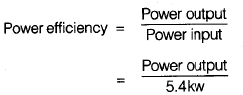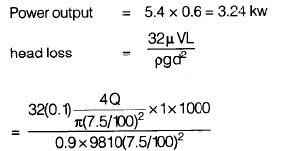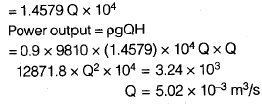Courses

# Fluid Mechanics - 1

## 15 Questions MCQ Test GATE Civil Engineering (CE) 2022 Mock Test Series | Fluid Mechanics - 1

Description
This mock test of Fluid Mechanics - 1 for GATE helps you for every GATE entrance exam. This contains 15 Multiple Choice Questions for GATE Fluid Mechanics - 1 (mcq) to study with solutions a complete question bank. The solved questions answers in this Fluid Mechanics - 1 quiz give you a good mix of easy questions and tough questions. GATE students definitely take this Fluid Mechanics - 1 exercise for a better result in the exam. You can find other Fluid Mechanics - 1 extra questions, long questions & short questions for GATE on EduRev as well by searching above.
QUESTION: 1

### If the velocity distribution over a plate is given by u = 2/3 y – y2 which v is the velocity in m/s at a distance y meter above the plate. The value of shear stress at y = 0 and y = 0.15m. Take dynamic viscosity of fluid as 8.63 poise.

Solution: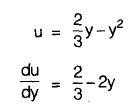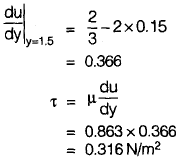QUESTION: 2

### A globe valve is the most suitable for applications, in which

Solution:

Butterfly valve: Regulates discharge
Ball valve: Maintains constant level in elevated tank
Foot valve: used in end of suction pipe
Globe valve: Similar to water tap, thus control fluid flow. Flow change its direction 90 degree twice to increase the head loss.
Pilot valve: Control high pressure and is used in emergency and safety controls.

QUESTION: 3

### In the inclined manometer shown in figure below, the reservoir is large. Its surface may be assumed to remain at a fixed elevation. A is connected to a gas pipeline and the deflection noted on the inclined glass tube is 100 mm. Assuming θ = 30° and the manometric fluid as oil with specific gravity of 0.86, the pressure at A is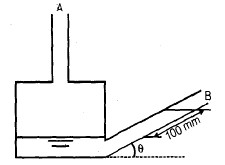Solution:

Total pressure at A will be equal to the pressure at the surface of manometric fluid because above the surface, the gas pipeline is connected.
Pressure at A = 100 sin 30° mm of oil = 50 mm of oil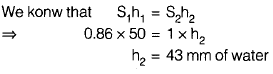QUESTION: 4

If flow velocity in x and y directions are given by u = 2x + 3y and v = –2y. The circulation around the circle of radius 2 units will be

Solution: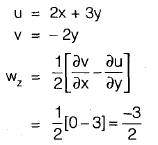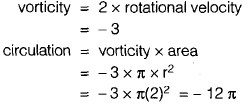QUESTION: 5

For a two dimensional flow,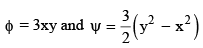Find the discharge passing between stream line passing through the points (1, 3) and (3, 3)

Solution: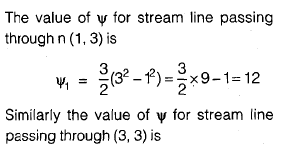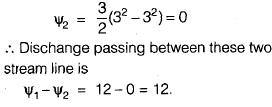QUESTION: 6

Through a very narrow gap of height ‘h’ a thin plate of large extent is pulled at a velocity ‘v’. On upper side of the plate is oil is viscosity u1 and the other side of oil of viscosity u2 . At what distance from the lower surface the plate should be placed so that pull required to drag the plate is minimum

Solution: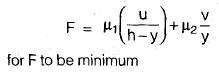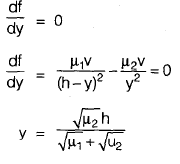QUESTION: 7

The compartment B and C shown in the figure below are closed and filled with air the barometer reads 99.572 kN/m2. When the gauges A and D reads as indicated what should be the value of x for gauge E. (mercury is the monometric liquid in each U tube gauge)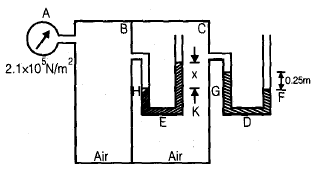Solution:

If P is the pressure intensity of air in compartment C in terms of obsolute unit then

QUESTION: 8

A cylinder 2.4 m diameter weights 2 kN and rests on the bottom of tank which is 1 m long. As shown in figure, water and oil are poured in to the left and right hand portions of the tank to depths 0.6 m and 1.2 m respectively. The magnitude of horizontal and vertical components of force which will keep the cylinder touching the tank at B will be respectively.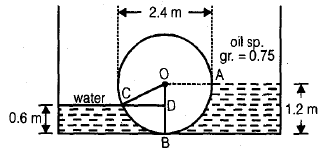Solution: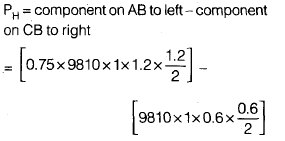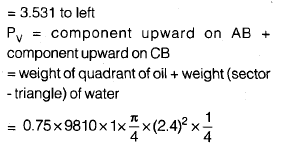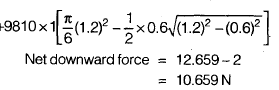QUESTION: 9

Consider the following

The components of velocity u and v along X and Y directions in a 2D flow problem of an incompressible fluid are

1. u = x2 cos y; v = – 2x sin y
2. u = x + 2; v = 1 – y
3. u = xyt; v = x3 – y2t/2
4. u = ln x + y; v = xy – y/x

Which of these will satisfy the continuity equation?

Solution: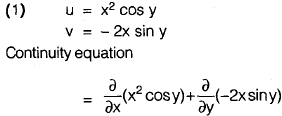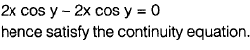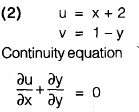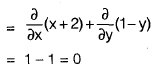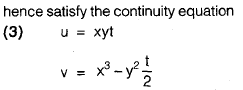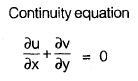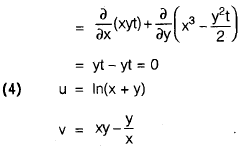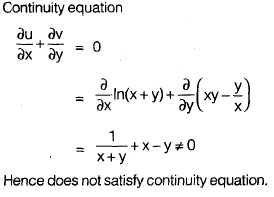QUESTION: 10

A venturimeter has its axis vertical, the inlet and throat diameter being 150 mm and 75 mm respectively. The throat is 225 mm above inlet. Petrol of specific gravity 0.78 flows up at a rate of 0.029 m3/s. The pressure difference between the inlet and throat will be (cd = 0.96 for venturimeter)

Solution: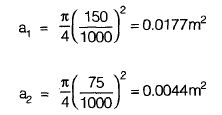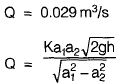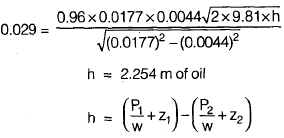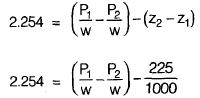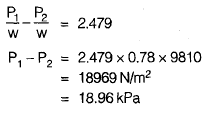QUESTION: 11

Water flows from a 300 mm pipe. Two pitot tubes are installed in the pipe. One on the centre line and other 75 mm from the centre line. If velocities at the two points are 3m/s and 2m/s respectively calculate the reading on the differential manometer connected to the two tubes

Solution: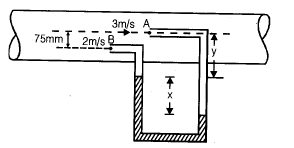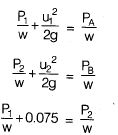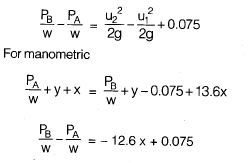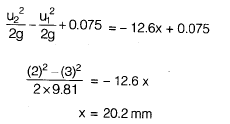QUESTION: 12

A pipe ABC connecting two reservoirs is 80 mm in diameter from A to B the pipe is horizontal as shown in the figure and from B to C if falls by 3.5 meters the length AB and BC are 25 m and 15 m respectively. If the water in the presence at A is 4m above the centre line of pipe and at C 1 m above the centre line of pipe. Neglect the loss at bend but consider all other losses. Take f = 0.024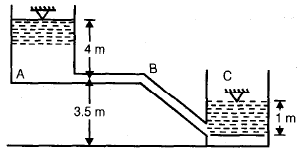Q.

Find the quantity of flow?

Solution: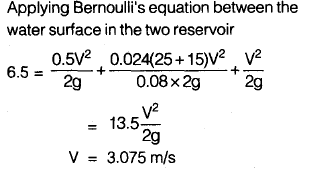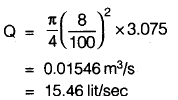QUESTION: 13

A pipe ABC connecting two reservoirs is 80 mm in diameter from A to B the pipe is horizontal as shown in the figure and from B to C if falls by 3.5 meters the length AB and BC are 25 m and 15 m respectively. If the water in the presence at A is 4m above the centre line of pipe and at C 1 m above the centre line of pipe. Neglect the loss at bend but consider all other losses. Take f = 0.024Q.

The pressure head at B is

Solution: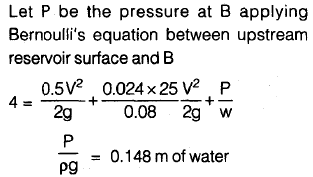QUESTION: 14

Air flows over a flat plate 1 m long at a velocity of 6 m/s. The sheer stress at the middle of plate will be

[Take S = 1.226 kg/m3, v = 0.15× 10–4 m3/s (0.15 strokes) for air]

Solution: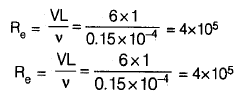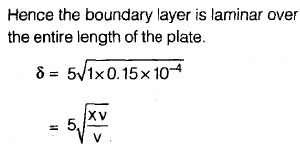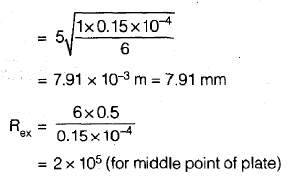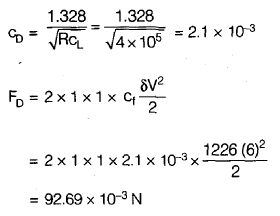QUESTION: 15

Oil is pumped through a 7.5 cm diameter pipe, 1 km long. The dynamic viscosity of oil is 0.1 N s/m2. A power input of 5.4 kW with an overall efficiency of 60% at the pump is noted. The discharge through the pump is (specific gravity of oil is 0.9)

Solution: